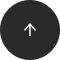PostgreSQL

# The Remainder in PostgreSQL, MS SQL Server, MySQL, and SQLite

#### Operators:

ABS, CASE WHEN, SIGN, %

#### Problem:

You want to find the (non-negative) remainder.

#### Example:

In the table `numbers`, you have two columns of integers: `a` and `b`.

ab
93
53
23
03
-23
-53
-93
5-3
-5-3
50
00

You want to compute the remainders from dividing `a` by `b`. Each remainder should be a non-negative integer value smaller than `b`.

#### Solution 1 (not entirely correct):

```SELECT
a,
b,
a % b AS remainder
FROM numbers;
```

The result is:

abremainder
930
532
232
030
-23-2
-53-2
-930
5-32
-5-3-2
50error
00error

#### Discussion:

This solution works correctly if a is non-negative. However, when it is negative, it doesn’t follow the mathematical definition of the remainder.

Conceptually, a remainder is what remains after an integer division of `a` by `b`. Mathematically, a remainder of two integers is a non-negative integer that is smaller than the divisor `b`. More precisely, it is a number r∈{0,1,...,b - 1} for which there exists some integer k such that a = k * b + r.

This is exactly how `a % b` works for the non-negative dividends in the column `a`:

`5 = 1 * 3 + 2`, so the remainder of 5 and 3 equals `2`.

`9 = 3 * 3 + 0`, so the remainder of 9 and 3 equals `0`.

`5 = (-1) * (-3) + 2`, so the remainder of 5 and -3 equals `2`.

Obviously, an error is shown if the divisor `b` is `0`, because you can't divide by `0`.

Getting the correct remainder is problematic when the dividend `a` is a negative number. Unfortunately, `a % b` can return a negative value when `a` is negative. E.g.:

`-2 % 5` returns `-2` when it should return `3`.

`-5 % -3` returns `-2` when it should return `1`.

#### Solution 2 (correct for all numbers):

```SELECT
a,
b,
CASE WHEN a % b >= 0
THEN a % b
ELSE
a % b + ABS(b)
END AS remainder
FROM numbers;
```

The result is:

abremainder
930
532
232
030
-231
-531
-930
5-32
-5-31
50error
00error

#### Discussion:

To compute the remainder of a division of any two integers (negative or non-negative), you can use the `CASE WHEN` construction. If `a % b `is non-negative, the remainder is simply `a % b`. Otherwise, we need to correct the result returned by `a % b`.

If `a % b` returns a negative value, you should add the absolute value of a divisor to `a % b`. That is, make it `a % b + ABS(b)`:

`-2 % 5` returns `-2` when it should return `3`. You can fix this by adding `5`.

`-5 % (-3)` returns `-2` when it should return `1`. You can fix this by adding `3`.

When `a % b` returns a negative value, the `CASE WHEN` result should be `a % b + ABS(b)`. This is how you get Solution 2. If you need a refresher on how the `ABS()` function works, take a look at the cookbook How to compute an absolute value in SQL.

Of course, if `b = 0`, you'll still get an error.

#### Solution 3 (correct for all numbers):

```SELECT
a,
b,
a % b + ABS(b) * (1 - SIGN(a % b + 0.5)) / 2 AS remainder
FROM numbers;
```

The result is:

abremainder
930
532
232
030
-231
-531
-930
5-32
-5-31
50error
00error

#### Discussion:

There is another way to solve this problem. Instead of a `CASE WHEN`, use a more complex one-line mathematical formula:

`a % b + ABS(b) * (1 - SIGN(a % b + 0.5)) / 2`

In Solution 2, `a % b + ABS(b)` was returned for cases when `a % b < 0`. Note that `a % b + ABS(b) = a % b + ABS(b) * 1 when a % b < 0`.

So, we can multiply `ABS(b)` by an expression that equals 1 for negative values of `a % b` and `0` for non-negative values of `a % b`. Since `a % b` is always an integer, the expression `a % b + 0.5` is always positive for `a % b >= 0` and negative for `a % b < 0`. You can use any positive number less than `1` instead of `0.5`.

The sign function `SIGN()` returns `1` if its argument is strictly positive, `-1` if it is strictly negative, and `0` if it equals `0`. However, you need something that returns only `0` and `1`, not `1` and `-1`. But no worries! Here’s how you fix this:

`(1 - 1) / 2 = 0`

`(1 - (-1)) / 2 = 1`

Then, the correct expression by which you should multiply `ABS(b)` is:

`(1 - SIGN(a % b + 0.5)) / 2`

So, the entire formula is:

`a % b + ABS(b) * (1 - SIGN(a % b + 0.5)) / 2`

#### Recommended articles: